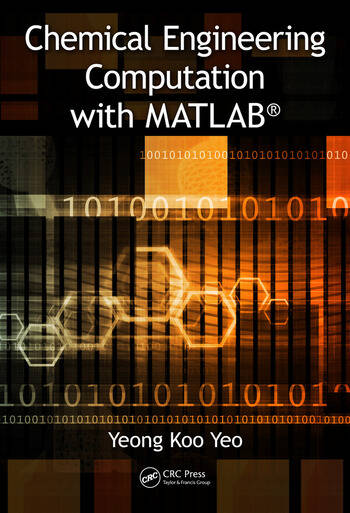# Chemical Engineering Computation with MATLAB®

## 1st Edition

Yeong Koo Yeo

CRC Press
Published August 7, 2017
Textbook - 608 Pages - 20 Color & 182 B/W Illustrations
ISBN 9781138039896 - CAT# K32976

For Instructors Request Inspection Copy

was \$135.00

USD\$108.00

SAVE ~\$27.00

FREE Standard Shipping!

## Preview

### Summary

Most problems encountered in chemical engineering are sophisticated and interdisciplinary. Thus, it is important for today’s engineering students, researchers, and professionals to be proficient in the use of software tools for problem solving. MATLAB® is one such tool that is distinguished by the ability to perform calculations in vector-matrix form, a large library of built-in functions, strong structural language, and a rich set of graphical visualization tools. Furthermore, MATLAB integrates computations, visualization and programming in an intuitive, user-friendly environment.

Chemical Engineering Computation with MATLAB® presents basic to advanced levels of problem-solving techniques using MATLAB as the computation environment. The book provides examples and problems extracted from core chemical engineering subject areas and presents a basic instruction in the use of MATLAB for problem solving. It provides many examples and exercises and extensive problem-solving instruction and solutions for various problems. Solutions are developed using fundamental principles to construct mathematical models and an equation-oriented approach is used to generate numerical results. A wealth of examples demonstrate the implementation of various problem-solving approaches and methodologies for problem formulation, problem solving, analysis, and presentation, as well as visualization and documentation of results. This book also provides aid with advanced problems that are often encountered in graduate research and industrial operations, such as nonlinear regression, parameter estimation in differential systems, two-point boundary value problems and partial differential equations and optimization.

#### Instructors

We provide complimentary e-inspection copies of primary textbooks to instructors considering our books for course adoption.